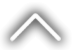2014 Annual Report
Ratios
 % 2014 2013 CAPITAL Capital Adequacy Ratio 18.2 13.2 Shareholders’ Equity/Total Assets 11.5 8.9 Shareholders’ Equity/(Total Assets+Non-cash Loans) 9.9 7.7 Shareholders’ Equity/(Deposits+Non-Deposit Resources) 13.6 10.1 ASSET QUALITY Total Loans/Total Assets 57.3 53.5 NPL (Gross)/Cash Loans 1.9 2.1 NPL (Gross)/(Non-cash Loans+Cash Loans) 1.5 1.7 FC Assets/FC Liabilities 95.2 95.4 LIQUIDITY Liquid Assets/Total Assets 13.1 14.0 Liquid Assets/(Deposits+Non-Deposit Resources) 15.4 16.0 PROFITABILITY Net Profit (Loss)/Average Total Assets 1.8 1.8 Net Profit (Loss)/Average Shareholders’ Equity 16.4 18.5 Net Profit (Loss)/Average Paid-in Capital 162.0 133.2 COST-INCOME STRUCTURE Interest Income/Interest Expenses 190.0 216.7 Non-Interest Income/Non-Interest Expenses 41.1 41.0 Total Income/Total Expenses 133.7 135.5 Interest Income/Total Income 88.4 86.0 Non-Interest Income/Total Income 11.6 14.0 Interest Expenses/Total Expenses 62.2 53.8 Non-Interest Expenses/Total Expenses 37.8 46.2